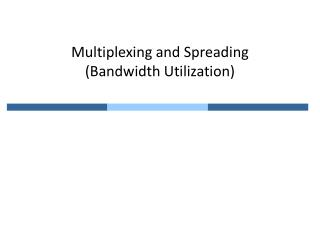Download PresentationMultiplexing and Spreading (Bandwidth Utilization)Multiplexing and Spreading (Bandwidth Utilization) - PowerPoint PPT Presentation

Download PresentationMultiplexing and Spreading (Bandwidth Utilization)
An Image/Link below is provided (as is) to download presentation

Download Policy: Content on the Website is provided to you AS IS for your information and personal use and may not be sold / licensed / shared on other websites without getting consent from its author. While downloading, if for some reason you are not able to download a presentation, the publisher may have deleted the file from their server.

- - - - - - - - - - - - - - - - - - - - - - - - - - - E N D - - - - - - - - - - - - - - - - - - - - - - - - - - -
Presentation Transcript

1. Multiplexing and Spreading(Bandwidth Utilization)

2. 1. Multiplexing • Multiplexing is the set of techniques that allow the simultaneous transmission of multiple signals across a single data link • Improves link efficiency by “sharing” • Categories • Frequency-Division Multiplexing (FDM) • Wavelength-Division Multiplexing (WDM) • Time-Division Multiplexing (TDM) • Code-Division Multiplexing (CDM)

3. Mux and Demux • Diving a link into multiple (n) channels using mux and demux

4. Frequency-Division Multiplexing (FDM) • Analog multiplexing technique that combines analog signals

5. FDM Muxing

6. FDM Demuxing

7. Example: Voice channel • We want to combine three voice channels into a link • Each voice channel occupies 4 kHz • Link has a bandwidth of 12 kHz, from 20 to 32 kHz • Modulate each of the three voice channels to a different bandwidth

8. Hierarchical Muxing 60 ~ 108 KHz. 312 ~ 552 KHz The first multiplexing step combines 12 voice inputs into a basic group, which is formed by having the nth input modulate a carrier at frequency fc= 60 + 4n KHz, where n = 1,2, …, 12. The next step in the FDM hierarchy involves the combination of five basic groups into a supergroup. This is accomplished by using the nth group to modulate a carrier of frequency fc= 372 + 48nKHz, where n= 1, 2, …, 5.

9. Guard band • Example: muxing of five (100kHz) channels

10. Wavelength-Division Multiplexing (WDM) • Is a technology which multiplexes a number of optical carrier signals onto a single optical fiber by using different wavelengths (i.ecolours) of laser light.

11. Prisms for Mulxing and Demuxing

12. Time-Division Multiplexing (TDM) • Digital multiplexing combining multiple low rate channels into a single high rate channel

13. Synchronous TDM • Link has a speed-up of n to combine n channels

14. TDM Example 1 • Synchronous TDM system • Combine four 1 Mbps streams • Unit of data is 1 bit • Questions • Input bit duration? • Output bit duration? • Output bit rate? • Output frame rate?

15. Questions • Input bit duration? • Output bit duration? • Output bit rate? • Output frame rate? 1 bit / 1Mbps = 1 μs 1/4 μs 4 Mbps 1 M frames / s

16. Multiple Multiplexing What if the data rates are not the same among inputs? • Multi-level Multiplexing • Multiple-slot Multiplexing When the data rate is a multiple of others.

17. Empty Slot and Stuffing • Empty slot • Stuffing When the data rate are not multiple integers of each others.

18. Frame Synchronization • Synchronization between the multiplexer and demultiplexer is a major issue. • If not synchronized, a bit belonging to one channel may be received by a wrong channel. • Additional information is need: frame bits

19. TDM Example 2 • Synchronous TDM system • Combine four 250 characters/s streams • Unit of data is 1 character (8 bits) • 1 synchronization bit is added to each frame • Questions • Input character duration? • Output frame rate? • Output frame duration? • Output bit rate? 1 / 250 = 4 ms 250 frames / s 1 / 250 = 4 ms 33 x 250 bits / s * Each frame has 33 (= 4 x 8 + 1) bits

20. Multiplexing Hierarchy • Telephone system (US)

21. T-1 Line Multiplexing

22. European Telephone System

23. Statistical TDM • Inefficiency from reserving time slots • Some inputs have high rate, some have low rate • Some inputs’ rate changes across time • Dynamic slot allocation for high efficiency

24. Comparison between synchronous TDM and statistical TDM Addressing for each line

25. 2. Spread Spectrum • Combine signals from different sources to fit into a larger bandwidth, but prevent eavesdropping and jamming • For this, spread spectrum techniques add redundancy • Two well-known methods • Frequency Hopping Spread Spectrum (FHSS) • Direct Sequence Spread Spectrum Synchronous (DSSS)

26. Idea of Spread Spectrum • Use more bandwidth

27. FHSS System • Frequency hopping spread spectrum (at sender)

28. Frequency Selection • Time is divided into cycles of the same length, each cycle has multiple periods (called “hop period”) • Different frequency is used at a different period • Each cycle has the same pattern of frequencies • The patterns are known to both sender and receiver ** pattern during a cycle

29. FHSS Cycles • Frequency usage i.e., time

30. FDM vs. FHSS • Recall the reasons why we spread signals • Security issues – eavesdropping, and jamming

31. DSSS System • Direct Sequence Spread Spectrum Synchronous (at sender)

32. Spread Signal of DSSS • At each period, codes change instead of freq. • To decode, one should know the seq. of codes

33. Homework • Exercise in Chap. 6 • 16 • 18 (Assume that the system can support 6 active sources simultaneously.) • 23